Function Repository Resource:

# InvolutionCount

Count the number of involutions

Contributed by: Wolfram Staff (original content by Sriram V. Pemmaraju and Steven S. Skiena)
 ResourceFunction["InvolutionCount"][n] gives the number of involutions on n elements.

## Details and Options

An involution on n elements is a permutation of length n that is its own inverse, i.e. a permutation σ with Length[σ]==n such that σ2 is the identity permutation.
Involution numbers are also known as telephone numbers, as they count the ways n telephone lines can be connected to each other with each line connecting to at most one other line.

## Examples

### Basic Examples (1)

Compute the number of involutions for n<10 (OEIS A000085):

 In:=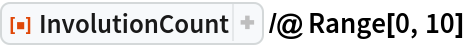Out=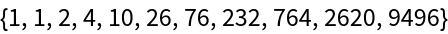### Properties and Relations (1)

InvolutionCount can be computed by the following recurrence relation due to Rothe:

 In:=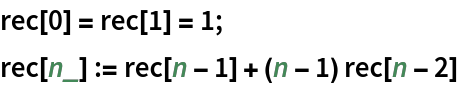In:=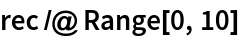Out=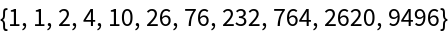In:=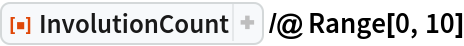Out=## Version History

• 1.0.0 – 29 May 2020